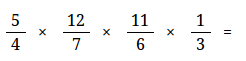### Fraction worksheet generator updated

I know this doesn't interest everyone... : ) It has to do with the fraction worksheet generator at HomeschoolMath.net.  I have worked on it in preparation for the release of the new version of Math Mammoth grade 6.

A new feature is that you can now make problems with 3 or 4 fractions, like this:(Add and subtract 3 unlike fractions)

or multiplications like this:(Multiply four fractions worksheet)

Then, you can use negative fractions (think pre-algebra / algebra 1) and practice addition:or subtraction:(or multiplication or division).

Try them here: Add or subtract negative fractions worksheet

Another big change is that now you can choose addition/subtraction (instead of just one operation), which then generates both addition and subtraction problems on the same sheet, and in the case of 3 or 4 fractions, includes both addition and subtraction in the problem.

Of course, you can generate regular addition/subtraction/multiplication/division problems with fractions and/or mixed numbers. Options are many and include limiting the numbers used in the problems to like fractions, proper fractions, and simplified fractions (in lowest terms).

You can also make missing number problems or force the answers to be whole numbers.

The worksheet generator page also has some ready-made worksheets for you to explore.